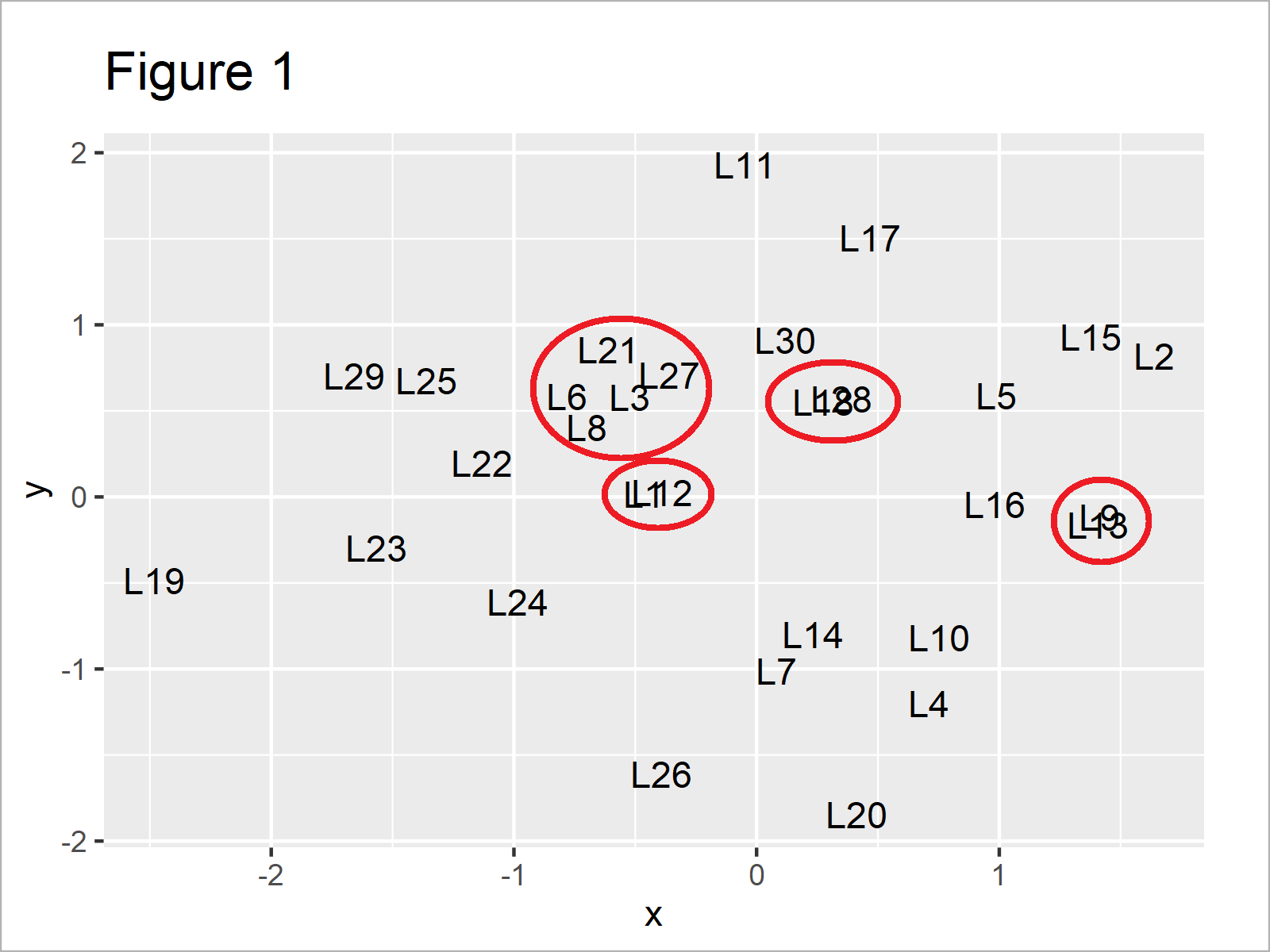# Avoid Overlap of Text Labels in ggplot2 Plot in R (Example)

This article illustrates how to remove overlap for geom_text labels in a ggplot2 graphic in the R programming language.

You’re here for the answer, so let’s get straight to the example.

## Example Data, Add-On Packages & Default Plot

Have a look at the following example data:

```set.seed(77358647)                   # Set seed
data <- data.frame(x = rnorm(30),    # Create example data
y = rnorm(30),
label = paste0("L", 1:30))Table 1 shows the head of the example data: Furthermore, it gets visible that our data consists of three columns. The variables x and y have the numeric class and the variable label is a character.

We also need to install and load the ggplot2 package, in order to apply the functions that are contained in the package:

```install.packages("ggplot2")          # Install & load ggplot2
library("ggplot2")```

Now, we can draw our data as follows.

```ggplot(data) +                       # Draw ggplot2 plot with labels
geom_text(aes(x, y, label = label))```After running the previous R programming syntax the ggplot2 plot with labels shown in Figure 1 has been drawn.

As you can see, some of the text labels created with the geom_text function are overlapping.

Next, I’ll show how to change that!

## Example: Avoid Overlap of ggplot2 Text Labels Using geom_text_repel() Function of ggrepel Package

In this example, I’ll demonstrate how to remove the overlap from text labels in a ggplot2 plot.

We first need to install and load the ggrepel package, to be able to use the functions that are included in the package:

```install.packages("ggrepel")          # Install ggrepel package

In the next step, we can use the geom_text_repel function instead of the geom_text function to avoid any text label overlap:

```ggplot(data) +                       # Draw labels without overlap
geom_text_repel(aes(x, y, label = label))```As shown in Figure 2, we have created a new ggplot2 plot where none of the text labels are overlapping.

## Video, Further Resources & Summary

Do you need further info on the R programming codes of this tutorial? Then I can recommend having a look at the following video instruction on my YouTube channel. In the video, I’m showing the topics of this article:

Please accept YouTube cookies to play this video. By accepting you will be accessing content from YouTube, a service provided by an external third party.If you accept this notice, your choice will be saved and the page will refresh.

Furthermore, you might have a look at the other RStudio tutorials on my website. You can find a selection of articles that are related to the removal of overlap for geom_text labels in a ggplot2 graphic below.

This tutorial has shown how to avoid overlap for geom_text labels in a ggplot2 plot in the R programming language. Please let me know in the comments, if you have further comments or questions. In addition, please subscribe to my email newsletter for updates on the newest tutorials.

Subscribe to the Statistics Globe Newsletter